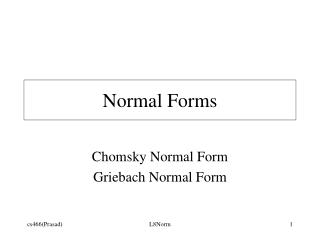DownloadDownload PresentationNormal Forms

Normal Forms

Télécharger la présentationNormal Forms

- - - - - - - - - - - - - - - - - - - - - - - - - - - E N D - - - - - - - - - - - - - - - - - - - - - - - - - - -
Presentation Transcript

1. Normal Forms Chomsky Normal Form Griebach Normal Form L8Norm

2. Language preserving transformations • Improve parsing efficiency • Prove properties about languages and derivations Shorter derivations L8Norm

3. Elimination of l-rules Reduces the length of the derivation L8Norm

4. Aim: Restrict the grammar such that • Approach: • Introduce S’ L8Norm

5. Add rules to capture the effect of l-rules to be deleted. (Ensures non-contracting rules) L8Norm

6. Example L8Norm

7. Determination of nullable non-terminals Bottom-up flow of information L8Norm

8. Algorithm Nullable Nonterminals NULL := {A | A->l eP}; repeat PREV := NULL; foreach A e V do if there is an A-rule A->w and we PREV* thenNULL := NULLU {A} untilNULL = PREV; L8Norm

9. Proof of correctness • Soundness • If A eNULL(final) then A=>* l. • Induction on the number of iterations of the loop. • Completeness • If A=>* l then A eNULL(final). • Induction on the minimal derivation of the null string from a non-terminal. • Termination • Bounded by the number of non-terminals. L8Norm

10. Elimination of Chainrules Removing renaming rules: redundant procedure calls. Top-down flow of information L8Norm

11. Construction of Chain(A) Chain(A) := {A}; PREV := f; repeat NEW := Chain(A) - PREV; PREV := Chain(A); foreach B e NEW do if there is a rule B->C thenChain(A) := Chain(A) U {C} untilChain(A) = PREV; L8Norm

12. Examples L8Norm

13. Elimination of useless symbols • A variable is useful if it occurs in a derivation that begins with the start symbol and generates a terminal string. • Reachable from S • Derives terminal string L8Norm

14. Construction of the set of variables that derive terminal string. • Bottom-up flow of information • Similar to the computation of nullable variables. • Construction of the set of variables that are reachable • Top-down flow of information • Similar to the computation of chained variables. L8Norm

15. Examples B does not derive terminal string; C unreachable. A unreachable. Empty set of productions “Non-termination” L8Norm

16. Chomsky Normal Form • A CFG is in Chomsky Normal Form if each rule is of the form: • Theorem: There is an algorithm to construct a grammar G’ in CNF that is equivalent to a CFG G. L8Norm

17. Construction • Obtain an equivalent grammar that does not contain l-rules, chain rules, and useless variables. • Apply following conversion on rules of the form: L8Norm

18. Significance of CNF • Length of derivation of a string of length n in CNF = (2n-1) (Cf. Number of nodes of a strictly binary tree with n-leaves) • Maximum depth of a parse tree = n • Minimum depth of a parse tree = L8Norm

19. Removal of direct left recursion • Causes infinite loop in top-down (depth-first) parsers. • Approach: Generate string from left to right. L8Norm

20. Note that absence of direct left recursion does not imply absence of left recursion. L8Norm

21. (Cf. Gaussian Elimination) L8Norm

22. Griebach Normal Form (* Constructs terminal prefixes that facilitates discovery of dead-ends *) • A CFG is in Griebach Normal Form if each rule is of the form • Theorem: There is an algorithm to construct a grammar G’ in GNF that is equivalent to a CFG G. L8Norm

23. Analogy: solving linear simultaneous equations What are the values of x,y, and z? (Solving for z and then back substituiting.) L8Norm

24. Example: conversion to GNF Introducing terminals as first element on RHS Eliminating left recursion L8Norm

25. The size of the equivalent GNF can be large compared to the original grammar. • Example CFG has 5 rules, but the corresponding GNF has 24 rules!! • Length of the derivation in GNF = Length of the string. • GNF is useful in relating CFGs (“generators”) to pushdown automata (“recognizers”/”acceptors”). L8Norm